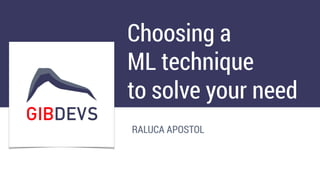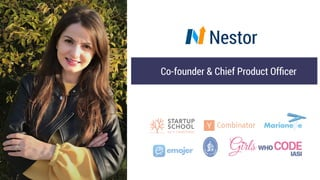Successfully reported this slideshow.

# Choosing a Machine Learning technique to solve your need×

1 of 52
1 of 52

# Choosing a Machine Learning technique to solve your need

Machine Learning extensive introduction presented by Raluca Aposton in GibDevs meetup April 2020

Machine Learning extensive introduction presented by Raluca Aposton in GibDevs meetup April 2020

## More Related Content

### Choosing a Machine Learning technique to solve your need

1. 1. Choosing a ML technique to solve your need RALUCA APOSTOL
2. 2. Nestor Co-founder & Chief Product Ofﬁcer
3. 3. Passion for data - science, analysis & interpretation
4. 4. Our chat today :) • What is Machine Learning? • What are some popular approaches? • How to think? • How to build your model? • What are the resources? • Let’s get to action.
5. 5. Artiﬁcial Intelligence • Anything which is not natural and created by humans is artiﬁcial. • Intelligence means ability to understand, reason, plan, adapt, etc. • So any code, tech or algorithm that enable machine to mimic, develop or demonstrate the human cognition or behavior is AI
6. 6. Applications for AI
7. 7. AI vs. ML
8. 8. AI vs. ML
9. 9. Connecting the dots …
10. 10. Connecting the dots …
11. 11. So what is Machine Learning? • Machine learning is the ﬁeld of study that gives computers the ability to learn without being explicitly programmed. • In simple term, Machine Learning means making prediction based on data.
12. 12. The ML Mindset “ML changes the way you think about a problem. The focus shifts from a mathematical science to a natural science, running experiments and using statistics, not logic, to analyze its results." – Peter Norvig Google Research Director
13. 13. The ML Mindset
14. 14. The ML Mindset F(a,b) = a + b a = 1; b = 2; 3
15. 15. The ML Mindset F(a,b) = a + b a = 1; b = 2; 3 F(a,b) = x*a + y*b a = 1; b = 2; 3 x = ?; y = ?
16. 16. The steps • Machine learning is part art and part science. :) • There is no one solution or one approach that ﬁts all. • There are several factors that can affect your decision to choose a machine learning algorithm.
17. 17. The steps
18. 18. Know your data Before you start looking at different ML algorithms, you need to have a clear picture of your data, your problem and your constraints.
19. 19. Know your data Before you start looking at different ML algorithms, you need to have a clear picture of your data, your problem and your constraints.
20. 20. Know your data 1. Look at Summary statistics and visualizations • Percentages can help identify the range for most of the data • Averages and medians can describe central tendency • Correlations can indicate strong relationships 2. Visualize the data • Box plots can identify outliers • Density plots and histograms show the spread of data • Scatter plots can describe bivariate relationships
21. 21. Since the collected data may be in an undesired format, unorganized, or extremely large, further steps are needed to enhance its quality. The three common steps for preprocessing data are formatting, cleaning, and sampling. Clean your data
22. 22. 1. How do I deal with missing value? • Missing data affects some models more than others. • Even for models that handle missing data, they can be sensitive to it (missing data for certain variables can result in poor predictions) 2. Does the data needs to be aggregated? Clean your data
23. 23. Clean your data
24. 24. Clean your data
25. 25. 3. What do I do with outliers? • Outliers can be very common in multidimensional data. • Some models are less sensitive to outliers than others. Usually tree models are less sensitive to the presence of outliers. However regression models, or any model that tries to use equations, could deﬁnitely be effected by outliers • Outliers can be the result of bad data collection, or they can be legitimate extreme values. Clean your data
26. 26. Sampling
27. 27. Augment your data 1. Feature engineering is the process of going from raw data to data that is ready for modeling. It can serve multiple purposes: • Make the models easier to interpret (e.g. binning) • Capture more complex relationships (e.g. NNs) • Reduce data redundancy and dimensionality (e.g. PCA) • Rescale variables (e.g. standardizing or normalizing) 2. Different models may have different feature engineering requirements.
28. 28. Categorize the problem 1. Categorize by input: • If you have labelled data, it’s a supervised learning problem. • If you have unlabelled data and want to ﬁnd structure, it’s an unsupervised learning problem. • If you want to optimize an objective function by interacting with an environment, it’s a reinforcement learning problem. 2. Categorize by output • If the output of your model is a number, it’s a regression problem. • If the output of your model is a class, it’s a classiﬁcation problem. • If the output of your model is a set of input groups, it’s a clustering problem. • Do you want to detect an anomaly ? That’s anomaly detection.
29. 29. Categorize the problem
30. 30. Understand your constraints • What is your data storage capacity? Depending on the storage capacity of your system, you might not be able to store gigabytes of classiﬁcation/regression models or gigabytes of data to clusterize. This is the case, for instance, for embedded systems. • Does the prediction have to be fast? In real time applications, it is obviously very important to have a prediction as fast as possible. For instance, in autonomous driving, it’s important that the classiﬁcation of road signs be as fast as possible to avoid accidents. • Does the learning have to be fast? In some circumstances, training models quickly is necessary: sometimes, you need to rapidly update, on the fly, your model with a different dataset.
31. 31. Choose the algorithm Identify the algorithms that are applicable and practical to implement using the tools at your disposal. Some of the factors affecting the choice of a model are: • Whether the model meets the business goals • How much preprocessing the model needs • How accurate the model is • How explainable the model is • How fast the model is: How long does it take to build a model, and how long does the model take to make predictions. • How scalable the model is
32. 32. Take care at complexity Making the same algorithm more complex increases the chance of overﬁtting. A model is more complex when: • It relies on more features to learn and predict (e.g. using two features vs ten features to predict a target) • It relies on more complex feature engineering (e.g. using polynomial terms, interactions, or principal components) • It has more computational overhead (e.g. a single decision tree vs. a random forest of 100 trees).
33. 33. ML Algorithms
34. 34. ML Algorithms
35. 35. Linear Regression Regression algorithms can be used for example, when you want to compute some continuous value as compared to Classiﬁcation where the output is categoric. • Time to go one location to another • Predicting sales of particular product next month • Impact of blood alcohol content on coordination • Predict monthly gift card sales and improve yearly revenue projections
36. 36. Logistic Regression Logistic regression performs binary classiﬁcation, so the label outputs are binary. It takes linear combination of features and applies non-linear function (sigmoid) to it, so it’s a very small instance of neural network. • Predicting the Customer Churn • Credit Scoring & Fraud Detection • Measuring the effectiveness of marketing campaigns
37. 37. Decision trees Single trees are used very rarely, but in composition with many others they build very efﬁcient algorithms such as Random Forest or Gradient Tree Boosting. • Investment decisions • Customer churn • Banks loan defaulters • Build vs Buy decisions • Sales lead qualiﬁcations
38. 38. K-means Sometimes you don’t know any labels and your goal is to assign labels according to the features of objects. This is called clusterization task. If there are questions like how is this organized or grouping something or concentrating on particular groups etc. in your problem statement then you should go with Clustering. • When there is a large group of users and you want to divide them into particular groups based on some common attributes.
39. 39. Principal component analysis Principal component analysis provides dimensionality reduction. Sometimes you have a wide range of features, probably highly correlated between each other, and models can easily overﬁt on a huge amount of data. Then, you can apply PCA.
40. 40. Support Vector Machines Support Vector Machine (SVM) is a supervised machine learning technique that is widely used in pattern recognition and classiﬁcation problems —  when your data has exactly two classes. • detecting persons with common diseases such as diabetes • hand-written character recognition • text categorization — news articles by topics • stock market price prediction
41. 41. Neural networks Neural Networks take in the weights of connections between neurons. Extremely complex models can be trained and they can be utilized as a kind of black box, without playing out an unpredictable complex feature engineering before training the model. Object recognition has been as of late enormously enhanced utilizing Deep Neural Networks. Applied to unsupervised learning tasks, such as feature extraction, deep learning also extracts features from raw images or speech with much less human intervention.
42. 42. Training & Evaluation
43. 43. Training & Evaluation
44. 44. Parameter Tuning
45. 45. Prediction
46. 46. What you need to know ? It is mandatory to learn a programming language, preferably Python, along with the required analytical and mathematical knowledge. You can touch on: • Linear algebra for data analysis: Scalars, Vectors, Matrices, and Tensors • Mathematical Analysis: Derivatives and Gradients • Probability theory and statistics • Multivariate Calculus • Algorithms and Complex Optimizations
47. 47. Programming Languages Python is hands down the best programming language for Machine Learning applications. Other programming languages that could to use for Machine Learning Applications are R, C++, JavaScript, Java, C#, Julia, Shell, TypeScript, and Scala.
48. 48. Cloud Support
49. 49. Test Interactive: • http://www.r2d3.us/visual-intro-to-machine-learning-part-1/ Test with Amazon SageMaker steps: 1. Create an AWS account 2. Launch a SageMaker Instance 3. Created a S3 bucket 4. Launch the Jupyter Notebook 5. Explore examples & tutorials
50. 50. Libraries • https://towardsdatascience.com/best-python-libraries-for-machine- learning-and-deep-learning-b0bd40c7e8c (Python) • https://blog.bitsrc.io/11-javascript-machine-learning-libraries-to-use- in-your-app-c49772cca46c (Javascript) • https://www.baeldung.com/java-ai (Java) • https://www.geeksforgeeks.org/machine-learning-in-c/ (C++)
51. 51. Datasets • https://towardsai.net/p/machine-learning/best-free-datasets-for- machine-learning-and-data-science/stanfordai/3451/ • https://towardsdatascience.com/free-data-sets-for-machine- learning-73e74554cc21 • https://www.ubuntupit.com/best-machine-learning-datasets-for- practicing-applied-ml/
52. 52. Nestor Co-founder & Chief Product Ofﬁcer Raluca Apostol raluca@nestorup.com